AT5. Periodic Trends

The Periodic Table and Periodic Trends

The Aufbau Process is a set of rules that allows us to predict the electronic configuration of an atom if we know how many electrons there are in the atom. If the periodic table is used as a tool, this process is pretty easy.

For atoms found in the first two columns of the periodic table (figure AT5.1), the configuration is a closed shell of core electrons, plus s electrons in a new shell. For example, potassium has a configuration [Ar]4s1. These atoms are often called the alkali and alkaline earth elements. Alkali elements, from the first column, have a configuration ending in s1; alkaline earth elements, from the second column, have configurations ending in s2. Together, these elements are often called the s-block elements, because their valence electrons are s electrons. Remember, the valence electrons are the ones beyond the noble gas core. In the case of potassium, they are the ones beyond [Ar].Figure AT5.1. The periodic table. Red elements are the alkali and alkaline earth metals (s-block). Yellow elements are the transition metals (d-block). Orange elements are the lanthanides and actinides (f-block). Green, blue and purple elements are the p-block; together with the s-block, they are called the main group elements. The main group is divided into metals (green), metalloids (teal), non-metals (blue) and noble or inert gases (purple).

The first two and the last six columns of the periodic table are called the main group elements. Alternatively, they are sometimes called the s-block and p-block elements, respectively. For example, phosphorus has a configuration, [Ne]4s24px1py1pz1, or simply [Ne]4s24p3.

The middle block of the periodic table consists of the transition metals or the d-block elements. For example, scandium has configuration [Ne]4s23d1.

The final two rows of the periodic table are the lanthanides and actinides. Collectively, they are called the f-block elements. Samarium, for example, is [Xe]6s24f6. These elements could really be inserted at the left-hand side of the d-block in the appropriate rows. Notice that lanthanum, element 57, is followed by hafnium, element 72, in the table. The element that really occurs next is element 58, cerium, and it is shown in the lanthanide row down below. The f-block elements are usually shown below in order to save space.

• The periodic table is divided into columns of atoms with similar electron configurations.
• Atoms with similar electron configurations have similar properties.

Chemical reactions depend on the movement of electrons. In a reaction, one atom may accept electrons from another atom. One atom may donate electrons to another atoms. The valence electrons are the outermost electrons in an atom; they are closest to the surface of an atom. That fact makes the valence electrons more likely to interact with other atoms. The valence are also the highest-energy electrons in an atom, and most likely to participate in a reaction.

For these reasons, atoms with similar electron configurations generally behave in similar ways. The repeating properties in each row of the periodic table, as observed by Mendeleev and others, reflect the repeating electron configurations in subsequent rows. The periodic table organizes atoms with similar configurations and properties together in columns.

Problem AT5.1.

For the following elements, suggest two other elements that would have similar properties.

1. zinc, Zn
2. calcium, Ca
3. oxygen, O
4. chlorine, Cl
5. chromium, Cr

Problem AT5.2.

Make a diagram showing the energy levels of different orbitals, arranged by principal quantum number."Periodic trends" refer to the way in which physical properties of atoms change across the periodic table. One of the most commonly used periodic trends in chemistry is electronegativity. Electronegativity is closely connected to the basic idea of chemical reactions: the transfer of an electron from one neutral atom to another. It refers to how strongly an atom attracts electrons from other atoms.

• Electronegativity is a measure of an atom's ability to draw electrons towards itself, or the ability of the nucleus to hold electrons tightly.

There are many scales of electronegativity, based on different physical measurements. Usually, electronegativity is set to an approximately 4-point scale. Atoms with electronegativity of around 4 draw electrons very strongly toward themselves. Atoms with electronegativity of 1 (or lower) only weakly draw electrons toward themselves.

The following data use the Allen scale of electronegativity. The Allen scale uses spectroscopic measurements to estimate the energy of valence electrons in an atom. From these values, the relative attraction of the atom for its valence electrons is placed on a 4 point scale (approximately).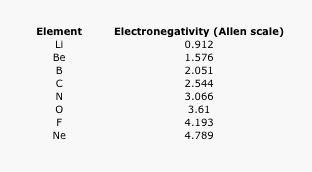Table AT5.1. The Allen electronegativity values of the second-row elements.

Some electronegativity scales do not have values for the noble gases, because they are based on experimental measurements of compounds, and noble gases do not commonly form compounds with other elements. Instead, they exist as single atoms. The Allen scale just depends on the ability of an atom to interact with light, which is something even noble gases can do. As a result, noble gases are also given electronegativity values on this scale. However, on many scales, fluorine would be the most electronegative atom here. As a result, fluorine is usually thought of as the most electronegative element.

Often it is useful to plot data on a graph. That way, we can get a better look at the relationship. For example, a quick glance at Figure AT5.2. shows that there is a smooth increase in electronegativity as we move across a row in the periodic table.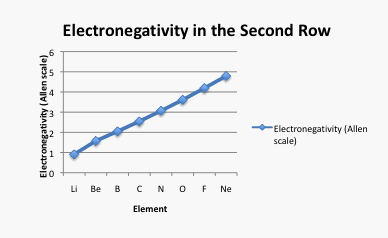Figure AT5.2. A plot of electronegativity versus atomic number in the second row of the periodic table.

Problem AT5.3.

Take a look at the graph in figure AT5.2. Can you explain why the electronegativity increases as atomic number increases?.

Problem AT5.4.

Suppose you need an electron. You have a boron atom and an oxygen atom. You try to take an electron away from one. Use Figure AT5.2. to predict which atom will give up the electron more easily.

Problem AT5.5.

Suppose you have an electron. You are able to send it into a vessel that contains a carbon atom and a fluorine atom. Use Figure AT5.2. to predict which atom is more likely to take the electron.

Problem AT5.6.

A covalent chemical bond is a pair of electrons shared between two atoms. Suppose you have a carbon-oxygen bond. Will the electrons be shared evenly between the two atoms, or will one atom pull the electrons more tightly towards itself? Use Figure AT5.2. to make your prediction.

What is happening as we move across a row in the periodic table? Why does electronegativity increase?

Keep in mind that the only difference from one element to the next is the number of protons in the nucleus. The number of protons is called the atomic number. If you know the number of protons you have, then you know what atoms you have. Electronegativity may have something to do with the number of protons in the nucleus. In fact, it should. The more protons there are in the nucleus, the more strongly electrons should be attracted to it. Each additional proton should add more electrostatic attraction for an electron. Fluorine, with nine protons, should attract electrons much more strongly than lithium, which has only three protons.

• Moving across a row of the periodic table, as protons are added to the nucleus, electrons are held more tightly.
• Electronegativity increases across a row in the periodic table.

It seems like that effect should be offset by the increasing number of electrons in the atom. Each time a proton is added, so is an electron. That electron should repel other electrons in the atom, cancelling out the effect of more protons in the nucleus.

However, the structure of the atom minimizes electron-electron repulsion a little bit. Remember that all the protons are in one place, the nucleus. All the electrons are a relatively great distance from the nucleus, in many different directions. Chances are, an additional electron is much farther away; it may be twice as far away as an additional proton in the nucleus. It may be all the way on the other side of the atom. Because electrons are spread out in the atom, and the distances between them is pretty large, the repulsive effect is a little smaller than the attractive effect of additional protons.

We can see that this trend is generally true across the periodic table, with a few exceptions here and there.Figure AT5.3. Electronegativity trends across the periodic table.

What happens as we move down a column in the periodic table? Table AT5.2. shows the Allen electronegativities of the alkali metals. These elements are also called the Group 1 elements or the Group IA elements. The "Group 1" designation is used because they are the first column or group in the periodic table. The data are also presented in Figure AT5.4.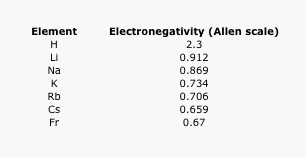Table AT5.2. The Allen electronegativity values of the alkali elements.Figure AT5.4. Plot of the Allen electronegativity values of the alkali elements.

There is a different trend here. In this case, lithium (atomic number 3) has more protons than hydrogen (atomic number 1). However, hydrogen is a lot more electronegative than lithium. Francium, with 87 protons in its nucleus, is the least electronegative alkali element.

Problem AT5.7.

Take a look at the graph in figure AT5.4. Can you explain why the electronegativity decreases as atomic number increases, going down this column?

Problem AT5.8.

An ionic chemical bond is a pair of ions attracted by their opposite charges. A cation is a positively charged ion; it may be an atom that has lost an electron. An anion is a negatively charged ion; it may be an atom that has gained an extra electron. Ions can form by moving an electron from one atom to another.

1. Suppose you have an ionic cesium-fluorine bond. Which ion is the cesium and which is the fluorine? Use Figure AT5.2 and Figure AT5.4. to make your prediction. Cesium is Cs.
2. Suppose you have an ionic sodium-oxygen bond. Which ion is the sodium and which is the oxygen? Use Figure AT5.2 and Figure AT5.4. to make your prediction. Sodium is Na (from the Latin, natrium).
3. Suppose you have an ionic potassium-hydrogen bond. Which ion is the potassium and which is the hydrogen? Use Figure AT5.2 and Figure AT5.4. to make your prediction. Potassium is K (from the Latin, kalium).

The biggest difference between two atoms in the same group in the periodic table is the principal quantum number. Remember, that corresponds to the "valence shell." Think of electrons as forming layers around the nucleus. Electrons with principal quantum number one form a first layer. Those with principal quantum number 2 form a second layer, and so on. Each layer is further away from the nucleus. Remember, electrostatic attraction gets weaker as charges get further away from each other. As electrons get further from the nucleus, they are less tightly held.

• Moving down a column in the periodic table, valence electrons are held less tightly because they get further from the nucleus.
• Electronegativity decreases as we move down a column in the periodic table.

We can see this general size trend in the following periodic table. This table presents covalent radii, which are related to the sizes of the atoms (although not exactly the same; data on atomic radii are not available for all atoms, however).Figure AT5.5. Covalent radii of the atoms.

Problem AT5.9.

Why does electronegativity fall so sharply between hydrogen and lithium, and much more subtly between lithium and sodium?

Problem AT5.10.

Which atom, in the following pairs, is more electronegative?

1. magnesium, Mg, or calcium, Ca
2. lead, Pb, or tin, Sn
3. silver, Ag, or antimony, Sb
4. gallium, Ga, or arsenic, As
5. tungsten, W, or copper, Cu
6. thallium, Tl, or sulfur, S

Problem AT5.11.

Electron ionization is the energy that must be added in order to pull an electron away from an atom.

1. Why do you think energy has to be used to pull an electron away from an atom? What is holding the electron there?
2. Explain the general trend in ionization energies seen in the following table (a larger value means more energy must be added to remove a first electron from the atom).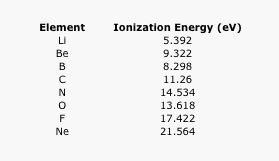Table AT5.6. The ionization energies of the second row elements.

Problem AT5.12.

Sometimes a plot of the data can be revealing. Ionization energies do not follow a smooth trend. Explain why it is a little easier to remove an electron from boron and oxygen than expected. (Electron configurations may be helpful here.)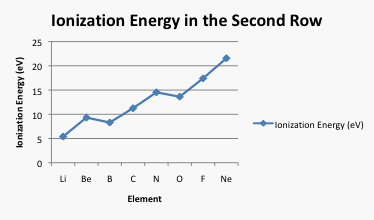Figure AT5.7. Plot of the ionization energies of the second row elements.

Problem AT5.13.

Explain the trend in the following data on ionization energy.Table AT5.4. The ionization energies of the alkali elements.

Problem AT5.14.

Electron affinity is the energy released when a free electron is picked up by an atom.

1. Why would energy be released when a free electron is taken by an atom?
2. Explain the general trend in the following electron affinity data.Table AT5.5. The electron affinities of the alkali elements.

Problem AT5.15.

1. Explain a general trend in the following electron affinity data.
2. There are several exceptions to the general trend. Why do beryllium and neon have such low electron affinities (almost zero)?
3. Nitrogen also has an electron affinity that is close to zero. Why?